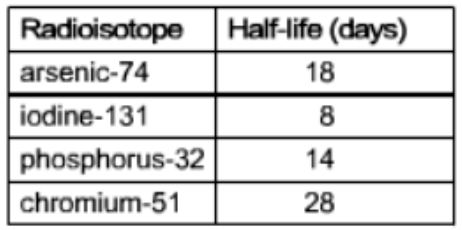# Problem: The half-lives of different medical radioisotopes are given in the table below. If the initial amount of arsenic-74 is 2.3 mCi, how much arsenic-74 (mCi) is left in the body after 54 days?

82% (42 ratings)
###### FREE Expert Solution

We’re being asked to determine the mass of arsenic–74 remaining after 54 days if there are 2.3 mCi (millicurie) of 74As initially.

Recall that radioactive/nuclear decay of isotopes follows first-order kinetics, and the integrated rate law for first-order reactions is:where:

[N]t = concentration at time t

k = decay constant

t = time

[N]0 = initial concentration

Also, recall that half-life is the time needed for the amount of a reactant to decrease by 50% or one-half

The half-life of a first-order reaction is given by:82% (42 ratings)###### Problem Details

The half-lives of different medical radioisotopes are given in the table below. If the initial amount of arsenic-74 is 2.3 mCi, how much arsenic-74 (mCi) is left in the body after 54 days?What scientific concept do you need to know in order to solve this problem?

Our tutors have indicated that to solve this problem you will need to apply the First Order Half Life concept. You can view video lessons to learn First Order Half Life. Or if you need more First Order Half Life practice, you can also practice First Order Half Life practice problems.

What is the difficulty of this problem?

Our tutors rated the difficulty ofThe half-lives of different medical radioisotopes are given ...as medium difficulty.

How long does this problem take to solve?

Our expert Chemistry tutor, Dasha took 6 minutes and 8 seconds to solve this problem. You can follow their steps in the video explanation above.

What professor is this problem relevant for?

Based on our data, we think this problem is relevant for Professor Hassell's class at BAYLOR.